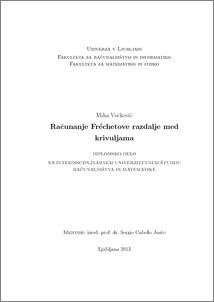# Computing the Fréchet distance between curves

Miha Vučkovič (2013) Computing the Fréchet distance between curves. EngD thesis.Preview
PDF

## Abstract

Comparing curves is an important and common problem in computer science. Curves are usually compared as sets of points, for example using the Hausdorff distance. Such an approach can lead to unrealistic results. Fréchet distance, which we explain in this thesis, is a natural measure of similarity between curves because it uses the curves in their entirety and respects their parametrization. There exists a discrete variant of the Fréchet distance which also respects the flow of the curves. In practice, this variant has a much better performance because of simpler primitive operations. The purpose of this thesis is to show the extension of algorithms for computing the Fréchet distance from polygonal curves to smooth curves, with some limitations. We provide concrete algorithms for solving the decision and optimization problems of Fréchet distance for smooth curves, provided some primitive operations are available. We prove that such algorithms make the same number of primitive operations as algorithms for polygonal curves: O(n^2) for the decision problem and O(n^2 log^2(n)) for the optimization problem, when the curves consist of n joined elementary pieces. We conclude that the running times of the primitive operations of these algorithms are too large to be useful in practice, and that the discrete variant of the Fréchet distance is still a better option.

Item Type: Thesis (EngD thesis)
Keywords: curves, Fréchet distance, parametric search
Number of Pages: 67
Language of Content: Slovenian
Mentor / Comentors:
Name and SurnameIDFunction
izr. prof. dr. Sergio Cabello Justo3487Mentor